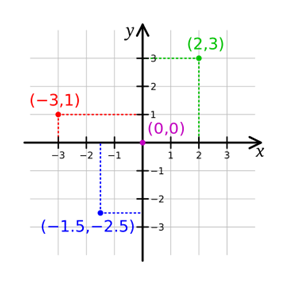# CBSE Class 9th Coordinate Geometry- Cartesian System

Here you will get the video lecture on topic 'Cartesian System' from CBSE Class 9 Mathematics chapter: Coordinate Geometry. Here, you will learn how to represent certain numbers on coordinate plane which is divided by the x and y axis. This video will help you develop a strong basis on cartesian system.

Nov 29, 2017 11:38 ISTCBSE Class 9 Maths: Cartesian System

In this video we will learn about the Cartesian System of Geometry in which the coordinate plane is divided by the x and y axis.

A Cartesian system is the one which specifies a point plotted uniquely on a plane by a pair of numerical coordinates, having a pre-defined distance from the two fixed perpendicular coordinates, measured in the same unit of length.• The numbers are represented on a number line on which the points are marked at equal distances.
• Two number lines are taken and intersected perpendicularly at the point zero also called the origin.
• The numbers on the right side of the horizontal number line, also x- axis, are positive and that on the left side are negative.
• The numbers to the top of the origin of the vertical number line, also y-axis, are positive and that below the origin are negative.
• The x- axis and the y-axis divide the coordinate plane into four parts called the quadrant.
• The quadrants are named in an anti-clockwise order, starting with I, II, III and IV.
• In the first quadrant both the x and y coordinates are positive
• In the second quadrant x coordinate is negative and the y coordinate is positive
• In the third quadrant both x and y coordinates are negative, and
• In fourth quadrant x coordinate is positive and the y coordinate is negative.
• Any point can be depicted on the coordinate plane by specifying its perpendicular distances from the x and y coordinates.